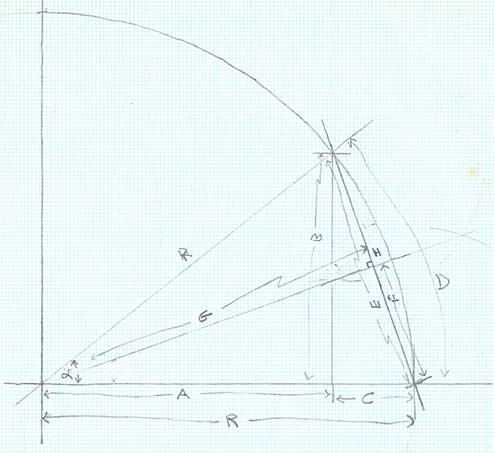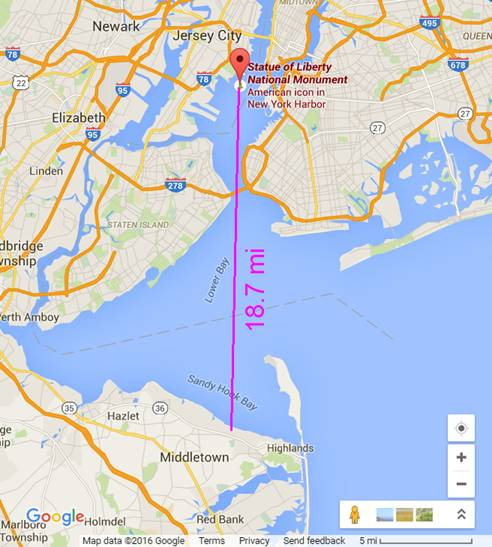Labels Values formula
R, radius of Earth (miles) 3963
D, distance from observer (miles) 18.7
α, angle to observer (radians) 0.004718647 D / R
A 3962.955881 R ∙ cos(α)
B 18.69993061 R ∙ sin(α)
C 0.044119272 R - A
E 18.69998265 sqrt(B²+C²)
F 9.349991326 E / 2
G 3962.98897 sqrt(R²-F²)
H 0.011029833 R - G
H in feet 58.2375203 H x 5280 ft/mi
Calculate the height of the effective barrier between observer and object under observation,
created by the curvature of the Earth, as a function of the distance between observer and
object under observation.  I.e., calculate the maximum height difference between a straight
line and a curve which follows the curvature of the Earth.The fundamental deficiency in this approach is the assumption that Earth has no atmosphere.
In the real world, the Earth's atmosphere acts as a lens, which refracts light. Depending
on atmospheric conditions, it is often possible for things beneath the horizon to appear at the
horizon, or even above the horizon. Here are a couple of very good web pages on this topic:
http://aty.sdsu.edu/~aty/explain/atmos_refr/horizon.html
http://aty.sdsu.edu/~aty/explain/atmos_refr/altitudes.htmlNote: the previous version of this spreadsheet (using 60 miles as the distance) is here: